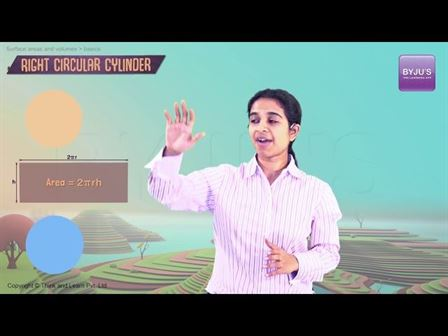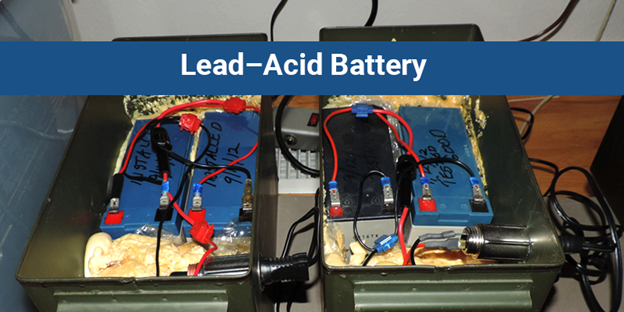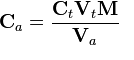# Area of Hollow CylinderA hollow cylinder is one which is empty from inside and has some difference between the internal and external radius. We see hollow cylinders every day in our day to day lives. Tubes, circular buildings, straws these are all examples of a hollow cylinder.## Area of a Hollow Cylinder:### Defining Terms:

Let ‘$\mathbf{r_{1}}$‘ be the outer radius of the given cylinder and ‘$\mathbf{r_{2}}$‘ be its inner radius and ‘$\mathbf{h}$‘ be its height.

$\mathbf{C_{1}}$‘ be the outer circumference and ‘$\mathbf{C_{2}}$‘ be the inner circumference.

L1 and L2 be the outer and inner surface areas respectively.

h be the height (or length) of a cylinder

t be the thickness of the cylinder ($\mathbf{r_{1}- r_{1}}$)

Area of Hollow Cylinder formulas:

The Circumference of a tcircle (C) is given by:

$C = 2\pi r$, therefore,$C_{1} = 2\pi r_{1}$$C_{2} = 2\pi r_{2}$

The Lateral Surface Area (L),for a cylinder is:

$L = C \times h = 2 \pi r h$, therefore,

$L_{1} = 2 \pi r_{1} h$, the external curved surface area

$L_{2} = 2 \pi r_{2} h$, the internal curved surface area

Thus Lateral Surface Area of a hollow cylinder = $L = 2 \pi r_{1} h + 2 \pi r_{2} h$

Cross sectional Area:

Let A be the area of a cross-section of a hollow cylinder,

A = $\pi r^{2}$, for a circle, therefore,

A1 = $\pi r_{1}^{2}$ for the area enclosed by $r_{1}$

A2 = $\pi r_{2}^{2}$ for the area enclosed by $r_{2}$

A = A1 – A2 for the cross sectional area of hollow cylinder

A = $\pi r_{1}^{2}- \pi r_{2}^{2} = \pi (r_{1}^{2}- r_{2}^{2})$

Total Surface Area of a Hollow Cylinder:

=$2 \pi h (R+ r) + 2 \pi (R^{2}- r^{2})$

=$2 \pi h (R+ r) + 2 \pi (R+ r) (R- r)$

=$2 \pi (R+ r) (h + R- r)$

Example: Find (in $cm^{2}$) the curved surface area of a hollow cylinder with thickness 2 cm external radius 8 cm and height is 20 cm.

Solution: Let the external radius, the internal radius and the height of the hollow cylinder be $r_{1}$, $r_{2}$ and h respectively.
$r_{2}$= 8-2 = 6 cm.

Curved surface area of a hollow cylinder = $2 \pi r_{1}h + 2 \pi r_{2}h$= $2 \pi h (r_{1} + r_{2}) = 2 \times \frac{22}{7} \times 20 (8+6)= 762.285 cm^{2}$